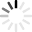Advertisement
Advertisement
Advertisement

# 7th Grade Math Quizzes & Trivia

Top Trending

A short maths quiz for the students of grade 7; It will cover questions from topics such as decimals, fractions, angle measures, percent, etc. So, take it and test your knowledge on the same.

Questions: 10  |  Attempts: 61662   |  Last updated: Oct 7, 2020
• Sample Question
You can have decimal oneths.This quiz is designed for 7th-grade students, which will test their essential mathematical skills on division, multiplication, fractions, decimals, Percents, and ratios. Please read the questions...

Questions: 8  |  Attempts: 50730   |  Last updated: Jul 2, 2020
• Sample Question
Name a equation that makes (+6), by using these numbers: (+9) (+7) (+2) (-8) (-3) (-1) !!Most people have a negative attitude when it comes to math but have no idea that it is the easiest subject one can come across and all someone needs to do is have some continuous practice. This quiz is about the math problems...

Questions: 21  |  Attempts: 14928   |  Last updated: Oct 1, 2020
• Sample Question
Evaluate for x = 11: x + 4The set of integers are numbers that represent whole things without pieces either above or below the zero line. When it comes to solving math problems on integers there are some rules that one has to keep in mind designed to help...

Questions: 10  |  Attempts: 15432   |  Last updated: Jul 30, 2020
• Sample Question
8+5=This quiz has been designed for 7th-grade students to test basic mathematical questions based on division, multiplication, fractions, decimals, Percents, and ratios. Please read the questions carefully and answer. So,...

Questions: 10  |  Attempts: 14510   |  Last updated: Feb 22, 2021
• Sample Question
What is 12*12?Advertisement

AdvertisementRelated
You May Also Like: 7th Grade Math Flashcards